# Stereographic projection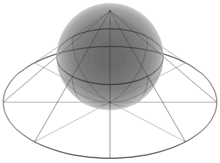3D illustration of a stereographic projection from the north pole onto a plane below the sphere

In geometry, the stereographic projection is a particular mapping (function) that projects a sphere onto a plane. The projection is defined on the entire sphere, except at one point: the projection point. Where it is defined, the mapping is smooth and bijective. It is conformal, meaning that it preserves angles. It is neither isometric nor area-preserving: that is, it preserves neither distances nor the areas of figures.

Intuitively, then, the stereographic projection is a way of picturing the sphere as the plane, with some inevitable compromises. Because the sphere and the plane appear in many areas of mathematics and its applications, so does the stereographic projection; it finds use in diverse fields including complex analysis, cartography, geology, and photography. In practice, the projection is carried out by computer or by hand using a special kind of graph paper called a stereographic net, shortened to stereonet, or Wulff net.

## History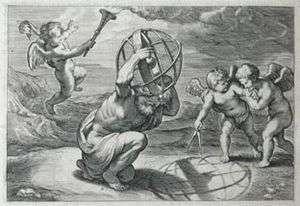Illustration by Rubens for "Opticorum libri sex philosophis juxta ac mathematicis utiles", by François d'Aguilon. It demonstrates how the projection is computed.

The stereographic projection was known to Hipparchus, Ptolemy and probably earlier to the Egyptians. It was originally known as the planisphere projection. Planisphaerium by Ptolemy is the oldest surviving document that describes it. One of its most important uses was the representation of celestial charts. The term planisphere is still used to refer to such charts.

In the 16th and 17th century, the equatorial aspect of the stereographic projection was commonly used for maps of the Eastern and Western Hemispheres. It is believed that already the map created in 1507 by Gualterius Lud was in stereographic projection, as were later the maps of Jean Roze (1542), Rumold Mercator (1595), and many others. In star charts, even this equatorial aspect had been utilised already by the ancient astronomers like Ptolemy.

François d'Aguilon gave the stereographic projection its current name in his 1613 work Opticorum libri sex philosophis juxta ac mathematicis utiles (Six Books of Optics, useful for philosophers and mathematicians alike).

In 1695, Edmond Halley, motivated by his interest in star charts, published the first mathematical proof that this map is conformal. He used the recently established tools of calculus, invented by his friend Isaac Newton.

## DefinitionStereographic projection of the unit sphere from the north pole onto the plane z = 0, shown here in cross section

This section focuses on the projection of the unit sphere from the north pole onto the plane through the equator. Other formulations are treated in later sections.

The unit sphere in three-dimensional space R3 is the set of points (x, y, z) such that x2 + y2 + z2 = 1. Let N = (0, 0, 1) be the "north pole", and let M be the rest of the sphere. The plane z = 0 runs through the center of the sphere; the "equator" is the intersection of the sphere with this plane.

For any point P on M, there is a unique line through N and P, and this line intersects the plane z = 0 in exactly one point P′. Define the stereographic projection of P to be this point P′ in the plane.

In Cartesian coordinates (x, y, z) on the sphere and (X, Y) on the plane, the projection and its inverse are given by the formulasIn spherical coordinates (φ, θ) on the sphere (with φ the zenith angle, 0 ≤ φ ≤ π, and θ the azimuth, 0 ≤ θ ≤ 2π) and polar coordinates (R, Θ) on the plane, the projection and its inverse areHere, φ is understood to have value π when R = 0. Also, there are many ways to rewrite these formulas using trigonometric identities. In cylindrical coordinates (r, θ, z) on the sphere and polar coordinates (R, Θ) on the plane, the projection and its inverse are## Properties

The stereographic projection defined in the preceding section sends the "south pole" (0, 0, −1) of the unit sphere to (0, 0), the equator to the unit circle, the southern hemisphere to the region inside the circle, and the northern hemisphere to the region outside the circle.

The projection is not defined at the projection point N = (0, 0, 1). Small neighborhoods of this point are sent to subsets of the plane far away from (0, 0). The closer P is to (0, 0, 1), the more distant its image is from (0, 0) in the plane. For this reason it is common to speak of (0, 0, 1) as mapping to "infinity" in the plane, and of the sphere as completing the plane by adding a "point at infinity". This notion finds utility in projective geometry and complex analysis. On a merely topological level, it illustrates how the sphere is homeomorphic to the one-point compactification of the plane.

In Cartesian coordinates a point P(x, y, z) on the sphere and its image P′(X, Y) on the plane either both are rational points or none of them: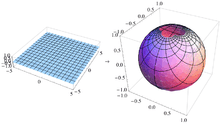A Cartesian grid on the plane appears distorted on the sphere. The grid lines are still perpendicular, but the areas of the grid squares shrink as they approach the north pole.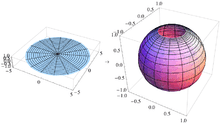A polar grid on the plane appears distorted on the sphere. The grid curves are still perpendicular, but the areas of the grid sectors shrink as they approach the north pole.

Stereographic projection is conformal, meaning that it preserves the angles at which curves cross each other (see figures). On the other hand, stereographic projection does not preserve area; in general, the area of a region of the sphere does not equal the area of its projection onto the plane. The area element is given in (X, Y) coordinates byAlong the unit circle, where X2 + Y2 = 1, there is no infinitesimal distortion of area. Near (0, 0) areas are distorted by a factor of 4, and near infinity areas are distorted by arbitrarily small factors.

The metric is given in (X, Y) coordinates byand is the unique formula found in Bernhard Riemann's Habilitationsschrift on the foundations of geometry, delivered at Göttingen in 1854, and entitled Über die Hypothesen welche der Geometrie zu Grunde liegen.

No map from the sphere to the plane can be both conformal and area-preserving. If it were, then it would be a local isometry and would preserve Gaussian curvature. The sphere and the plane have different Gaussian curvatures, so this is impossible.

The conformality of the stereographic projection implies a number of convenient geometric properties. Circles on the sphere that do not pass through the point of projection are projected to circles on the plane. Circles on the sphere that do pass through the point of projection are projected to straight lines on the plane. These lines are sometimes thought of as circles through the point at infinity, or circles of infinite radius.

All lines in the plane, when transformed to circles on the sphere by the inverse of stereographic projection, meet at the projection point. Parallel lines, which do not intersect in the plane, are transformed to circles tangent at projection point. Intersecting lines are transformed to circles that intersect transversally at two points in the sphere, one of which is the projection point. (Similar remarks hold about the real projective plane, but the intersection relationships are different there.)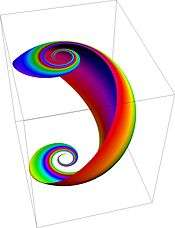The sphere, with various loxodromes shown in distinct colors

The loxodromes of the sphere map to curves on the plane of the formwhere the parameter a measures the "tightness" of the loxodrome. Thus loxodromes correspond to logarithmic spirals. These spirals intersect radial lines in the plane at equal angles, just as the loxodromes intersect meridians on the sphere at equal angles.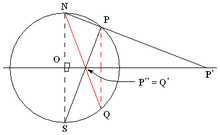The stereographic projection relates to the plane inversion in a simple way. Let P and Q be two points on the sphere with projections P′ and Q′ on the plane. Then P′ and Q′ are inversive images of each other in the image of the equatorial circle if and only if P and Q are reflections of each other in the equatorial plane.

In other words, if:

• P is a point on the sphere, but not a 'north pole' N and not its antipode, the 'south pole' S,
• P′ is the image of P in a stereographic projection with the projection point N and
• P″ is the image of P in a stereographic projection with the projection point S,

then P′ and P″ are inversive images of each other in the unit circle.## Wulff net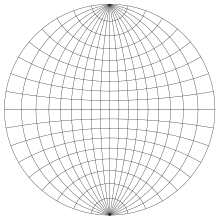Wulff net or stereonet, used for making plots of the stereographic projection by hand

Stereographic projection plots can be carried out by a computer using the explicit formulas given above. However, for graphing by hand these formulas are unwieldy. Instead, it is common to use graph paper designed specifically for the task. This special graph paper is called a stereonet or Wulff net, after the Russian mineralogist George (Yuri Viktorovich) Wulff.

To make a Wulff net, one places a grid of parallels and meridians on the hemisphere, and then stereographically projects these curves to the disk. Depending on the particular projection used, the parallels and meridians may or may not match those usually encountered in geography. For example, the figure at left is constructed using the conventions of the Definition section above. Because the projection point is (0, 0, 1), the Wulff net depicts the southern hemisphere z ≤ 0. The equator plots at the circular boundary of the Wulff net, and the south pole plots at the center of the Wulff net. The parallels are chosen to be small circles about the y-axis, and all of the meridians pass through (0, 1, 0) and (0, −1, 0).

In the figure, the area-distorting property of the stereographic projection can be seen by comparing a grid sector near the center of the net with one at the far right of the net. The two sectors have equal areas on the sphere. On the disk, the latter has nearly four times the area of the former. If one uses finer and finer grids on the sphere, then the ratio of the areas approaches exactly 4.

On the Wulff net, the images of the parallels and meridians intersect at right angles. This orthogonality property is a consequence of the angle-preserving property of the stereoscopic projection. (However, the angle-preserving property is stronger than this property. Not all projections that preserve the orthogonality of parallels and meridians are angle-preserving.)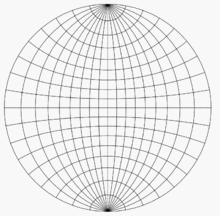Illustration of steps 1–4 for plotting a point on a Wulff net

For an example of the use of the Wulff net, imagine two copies of it on thin paper, one atop the other, aligned and tacked at their mutual center. Let P be the point on the lower unit hemisphere whose spherical coordinates are (140°, 60°) and whose Cartesian coordinates are (0.321, 0.557, −0.766). This point lies on a line oriented 60° counterclockwise from the positive x-axis (or 30° clockwise from the positive y-axis) and 50° below the horizontal plane z = 0. Once these angles are known, there are four steps to plotting P:

1. Using the grid lines, which are spaced 10° apart in the figures here, mark the point on the edge of the net that is 60° counterclockwise from the point (1, 0) (or 30° clockwise from the point (0, 1)).
2. Rotate the top net until this point is aligned with (1, 0) on the bottom net.
3. Using the grid lines on the bottom net, mark the point that is 50° toward the center from that point.
4. Rotate the top net oppositely to how it was oriented before, to bring it back into alignment with the bottom net. The point marked in step 3 is then the projection that we wanted.

To plot other points, whose angles are not such round numbers as 60° and 50°, one must visually interpolate between the nearest grid lines. It is helpful to have a net with finer spacing than 10°. Spacings of 2° are common.

To find the central angle between two points on the sphere based on their stereographic plot, overlay the plot on a Wulff net and rotate the plot about the center until the two points lie on or near a meridian. Then measure the angle between them by counting grid lines along that meridian.

## Other formulations and generalizationsStereographic projection of the unit sphere from the north pole onto the plane z = −1, shown here in cross section

Some authors define stereographic projection from the north pole (0, 0, 1) onto the plane z = −1, which is tangent to the unit sphere at the south pole (0, 0, −1). The values X and Y produced by this projection are exactly twice those produced by the equatorial projection described in the preceding section. For example, this projection sends the equator to the circle of radius 2 centered at the origin. While the equatorial projection produces no infinitesimal area distortion along the equator, this pole-tangent projection instead produces no infinitesimal area distortion at the south pole.

Other authors use a sphere of radius 1/2 and the plane z = −1/2. In this case the formulae becomeStereographic projection of a sphere from a point Q onto the plane E, shown here in cross section

In general, one can define a stereographic projection from any point Q on the sphere onto any plane E such that

• E is perpendicular to the diameter through Q, and
• E does not contain Q.

As long as E meets these conditions, then for any point P other than Q the line through P and Q meets E in exactly one point P′, which is defined to be the stereographic projection of P onto E.

All of the formulations of stereographic projection described thus far have the same essential properties. They are smooth bijections (diffeomorphisms) defined everywhere except at the projection point. They are conformal and not area-preserving.

More generally, stereographic projection may be applied to the n-sphere Sn in (n + 1)-dimensional Euclidean space En+1. If Q is a point of Sn and E a hyperplane in En+1, then the stereographic projection of a point PSn{Q} is the point P′ of intersection of the line QP with E. In Cartesian coordinates (xi, i from 0 to n) on the sphere and (Xi, i from 1 to n) on the plane, the projection from Q = (1, 0, 0, ..., 0) is given byDefiningthe inverse is given byStill more generally, suppose that S is a (nonsingular) quadric hypersurface in the projective space Pn+1. In other words, S is the locus of zeros of a non-singular quadratic form f(x0, ..., xn+1) in the homogeneous coordinates xi. Fix any point Q on S and a hyperplane E in Pn+1 not containing Q. Then the stereographic projection of a point P in S{Q} is the unique point of intersection of QP with E. As before, the stereographic projection is conformal and invertible outside of a "small" set. The stereographic projection presents the quadric hypersurface as a rational hypersurface. This construction plays a role in algebraic geometry and conformal geometry.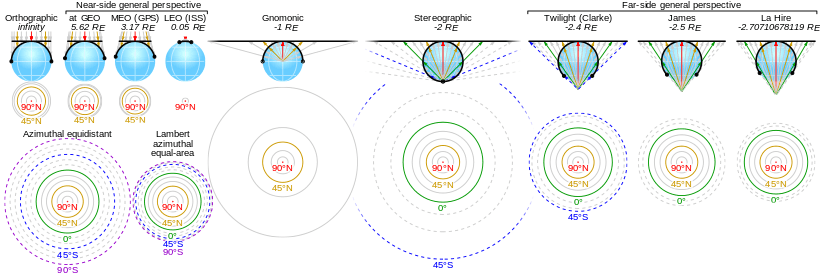Comparison of the Stereographic projection and some azimuthal projections centred on 90° N at the same scale, ordered by projection altitude in Earth radii. (click for detail)

## Applications within mathematics

### Complex analysis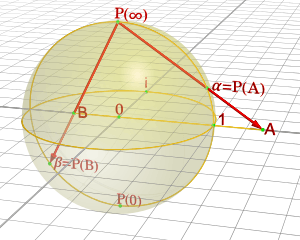The complex plane and the Riemann sphere above it

Although any stereographic projection misses one point on the sphere (the projection point), the entire sphere can be mapped using two projections from distinct projection points. In other words, the sphere can be covered by two stereographic parametrizations (the inverses of the projections) from the plane. The parametrizations can be chosen to induce the same orientation on the sphere. Together, they describe the sphere as an oriented surface (or two-dimensional manifold).

This construction has special significance in complex analysis. The point (X, Y) in the real plane can be identified with the complex number ζ = X + iY. The stereographic projection from the north pole onto the equatorial plane is thenSimilarly, letting ξ = X − iY be another complex coordinate, the functionsdefine a stereographic projection from the south pole onto the equatorial plane. The transition maps between the ζ- and ξ-coordinates are then ζ = 1/ξ and ξ = 1/ζ, with ζ approaching 0 as ξ goes to infinity, and vice versa. This facilitates an elegant and useful notion of infinity for the complex numbers and indeed an entire theory of meromorphic functions mapping to the Riemann sphere. The standard metric on the unit sphere agrees with the Fubini–Study metric on the Riemann sphere.

### Visualization of lines and planes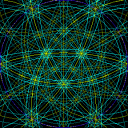Animation of Kikuchi lines of four of the eight <111> zones in an fcc crystal. Planes edge-on (banded lines) intersect at fixed angles.

The set of all lines through the origin in three-dimensional space forms a space called the real projective plane. This space is difficult to visualize, because it cannot be embedded in three-dimensional space.

However, one can "almost" visualize it as a disk, as follows. Any line through the origin intersects the southern hemisphere z  0 in a point, which can then be stereographically projected to a point on a disk. Horizontal lines intersect the southern hemisphere in two antipodal points along the equator, either of which can be projected to the disk; it is understood that antipodal points on the boundary of the disk represent a single line. (See quotient topology.) So any set of lines through the origin can be pictured, almost perfectly, as a set of points in a disk.

Also, every plane through the origin intersects the unit sphere in a great circle, called the trace of the plane. This circle maps to a circle under stereographic projection. So the projection lets us visualize planes as circular arcs in the disk. Prior to the availability of computers, stereographic projections with great circles often involved drawing large-radius arcs that required use of a beam compass. Computers now make this task much easier.

Further associated with each plane is a unique line, called the plane's pole, that passes through the origin and is perpendicular to the plane. This line can be plotted as a point on the disk just as any line through the origin can. So the stereographic projection also lets us visualize planes as points in the disk. For plots involving many planes, plotting their poles produces a less-cluttered picture than plotting their traces.

This construction is used to visualize directional data in crystallography and geology, as described below.

### Other visualization

Stereographic projection is also applied to the visualization of polytopes. In a Schlegel diagram, an n-dimensional polytope in Rn+1 is projected onto an n-dimensional sphere, which is then stereographically projected onto Rn. The reduction from Rn+1 to Rn can make the polytope easier to visualize and understand.

### Arithmetic geometry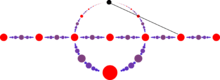The rational points on a circle correspond, under stereographic projection, to the rational points of the line.

In elementary arithmetic geometry, stereographic projection from the unit circle provides a means to describe all primitive Pythagorean triples. Specifically, stereographic projection from the north pole (0,1) onto the x-axis gives a one-to-one correspondence between the rational number points (x, y) on the unit circle (with y ≠ 1) and the rational points of the x-axis. If (m/n, 0) is a rational point on the x-axis, then its inverse stereographic projection is the pointwhich gives Euclid's formula for a Pythagorean triple.

### Tangent half-angle substitution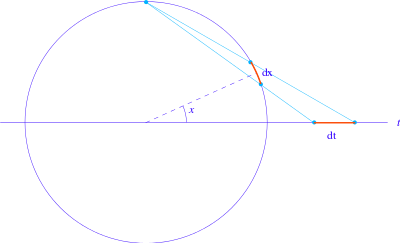The pair of trigonometric functions (sin x, cos x) can be thought of as parametrizing the unit circle. The stereographic projection gives an alternative parametrization of the unit circle:Under this reparametrization, the length element dx of the unit circle goes over toThis substitution can sometimes simplify integrals involving trigonometric functions.

## Applications to other disciplines

### Cartography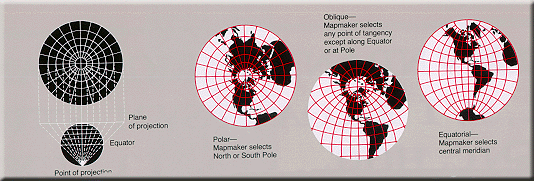Stereographic projection is used to map the Earth, especially near the poles, but also near other points of interest.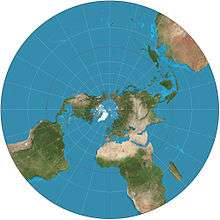Stereographic projection of the world north of 30°S. 15° graticule.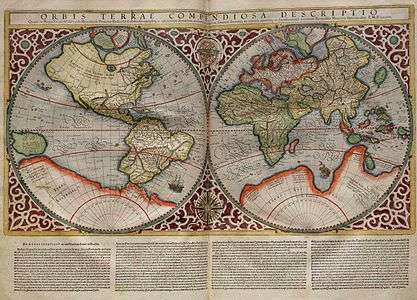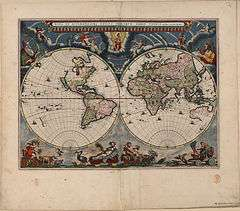The fundamental problem of cartography is that no map from the sphere to the plane can accurately represent both angles and areas. In general, area-preserving map projections are preferred for statistical applications, while angle-preserving (conformal) map projections are preferred for navigation.

Stereographic projection falls into the second category. When the projection is centered at the Earth's north or south pole, it has additional desirable properties: It sends meridians to rays emanating from the origin and parallels to circles centered at the origin.

The stereographic is the only projection that maps all circles of a sphere to circles. This property is valuable in planetary mapping when craters are typical features. The set of circles passing through the point of projection have unbounded radius, and therefore degenerate into lines.

### Crystallography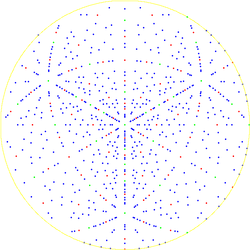A crystallographic pole figure for the diamond lattice in  direction
Main article: Pole figure

In crystallography, the orientations of crystal axes and faces in three-dimensional space are a central geometric concern, for example in the interpretation of X-ray and electron diffraction patterns. These orientations can be visualized as in the section Visualization of lines and planes above. That is, crystal axes and poles to crystal planes are intersected with the northern hemisphere and then plotted using stereographic projection. A plot of poles is called a pole figure.

In electron diffraction, Kikuchi line pairs appear as bands decorating the intersection between lattice plane traces and the Ewald sphere thus providing experimental access to a crystal's stereographic projection. Model Kikuchi maps in reciprocal space, and fringe visibility maps for use with bend contours in direct space, thus act as road maps for exploring orientation space with crystals in the transmission electron microscope.

### Geology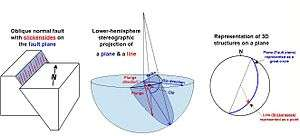Use of lower hemisphere stereographic projection to plot planar and linear data in structural geology, using the example of a fault plane with a slickenside lineation

Researchers in structural geology are concerned with the orientations of planes and lines for a number of reasons. The foliation of a rock is a planar feature that often contains a linear feature called lineation. Similarly, a fault plane is a planar feature that may contain linear features such as slickensides.

These orientations of lines and planes at various scales can be plotted using the methods of the Visualization of lines and planes section above. As in crystallography, planes are typically plotted by their poles. Unlike crystallography, the southern hemisphere is used instead of the northern one (because the geological features in question lie below the Earth's surface). In this context the stereographic projection is often referred to as the equal-angle lower-hemisphere projection. The equal-area lower-hemisphere projection defined by the Lambert azimuthal equal-area projection is also used, especially when the plot is to be subjected to subsequent statistical analysis such as density contouring.

### Photography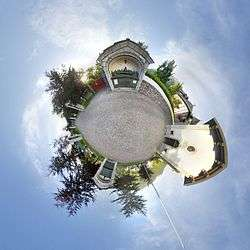Stereographic projection of the spherical panorama of the Last Supper sculpture by Michele Vedani in Esino Lario, Lombardy, Italy during Wikimania 2016

Some fisheye lenses use a stereographic projection to capture a wide-angle view. Compared to more traditional fisheye lenses which use an equal-area projection, areas close to the edge retain their shape, and straight lines are less curved. However, stereographic fisheye lenses are typically more expensive to manufacture. Image remapping software, such as Panotools, allows the automatic remapping of photos from an equal-area fisheye to a stereographic projection.

The stereographic projection has been used to map spherical panoramas. This results in effects known as a little planet (when the center of projection is the nadir) and a tube (when the center of projection is the zenith).

The popularity of using stereographic projections to map panoramas over other azimuthal projections is attributed to the shape preservation that results from the conformality of the projection.

## References

1. Snyder (1993).
2. According to (Snyder 1993), although he acknowledges he did not personally see it
3. Snyder (1989).
4. Brown, Lloyd Arnold : The story of maps, p.59.
5. According to (Elkins, 1988) who references Eckert, "Die Kartenwissenschaft", Berlin 1921, pp 121123
6. Timothy Feeman. 2002. "Portraits of the Earth: A Mathematician Looks at Maps". American Mathematical Society.
7. Wulff, George, Untersuchungen im Gebiete der optischen Eigenschaften isomorpher Kristalle: Zeits. Krist.,36, 1–28 (1902)
8. Cf. Apostol (1974) p. 17.
9. Gelfand, Minlos & Shapiro 1963
10. Cf. Pedoe (1988).
11. Cf. Shafarevich (1995).
12. M. von Heimendahl, W. Bell and G. Thomas (1964) Applications of Kikuchi line analyses in electron microscopy, J. Appl. Phys. 35:12, 36143616.
13. P. Fraundorf, Wentao Qin, P. Moeck and Eric Mandell (2005) Making sense of nanocrystal lattice fringes, J. Appl. Phys. 98:114308.
14. Samyang 8 mm f/3.5 Fisheye CS
15. "Samyang 8 mm f/3.5 Aspherical IF MC Fish-eye". lenstip.com. Retrieved 2011-07-07.
16. German et al. (2007).

### Sources

• Apostol, Tom (1974). Mathematical Analysis (2 ed.). Addison-Wesley. ISBN 0-201-00288-4.
• Brown, James & Churchill, Ruel (1989). Complex variables and applications. New York: McGraw-Hill. ISBN 0-07-010905-2.
• Casselman, Bill (2014), Feature column February 2014:Stereographic Projection, AMS, retrieved 2014-12-12
• German, Daniel; Burchill, L.; Duret-Lutz, A.; Pérez-Duarte, S.; Pérez-Duarte, E.; Sommers, J. (June 2007). "Flattening the Viewable Sphere". "Proceedings of Computational Aesthetics 2007". Banff: Eurographics. pp. 2328.
• Gelfand, I.M.; Minlos, R.A.; Shapiro, Z.Ya. (1963), Representations of the Rotation and Lorentz Groups and their Applications, New York: Pergamon Press
• Do Carmo; Manfredo P. (1976). Differential geometry of curves and surfaces. Englewood Cliffs, New Jersey: Prentice Hall. ISBN 0-13-212589-7.
• Elkins, James (1988). "Did Leonardo Develop a Theory of Curvilinear Perspective?: Together with Some Remarks on the 'Angle' and 'Distance' Axioms". Journal of the Warburg and Courtauld Institutes. The Warburg Institute. 51: 190196. doi:10.2307/751275. JSTOR 751275.
• Oprea, John (2003). Differential geometry and applications. Englewood Cliffs, New Jersey: Prentice Hall. ISBN 0-13-065246-6.
• Pedoe, Dan (1988). Geometry. Dover. ISBN 0-486-65812-0.
• Shafarevich, Igor (1995). Basic Algebraic Geometry I. Springer. ISBN 0-387-54812-2.
• Snyder, John P. (1987). Map Projections − A Working Manual, Professional Paper 1395. US Geological Survey.
• Snyder, John P. (1989). An Album of Map Projections, Professional Paper 1453. US Geological Survey.
• Snyder, John P. (1993). Flattening the Earth. University of Chicago. ISBN 0-226-76746-9.
• Spivak, Michael (1999). A comprehensive introduction to differential geometry, Volume IV. Houston, Texas: Publish or Perish. ISBN 0-914098-73-X.Wikimedia Commons has media related to Stereographic projection.